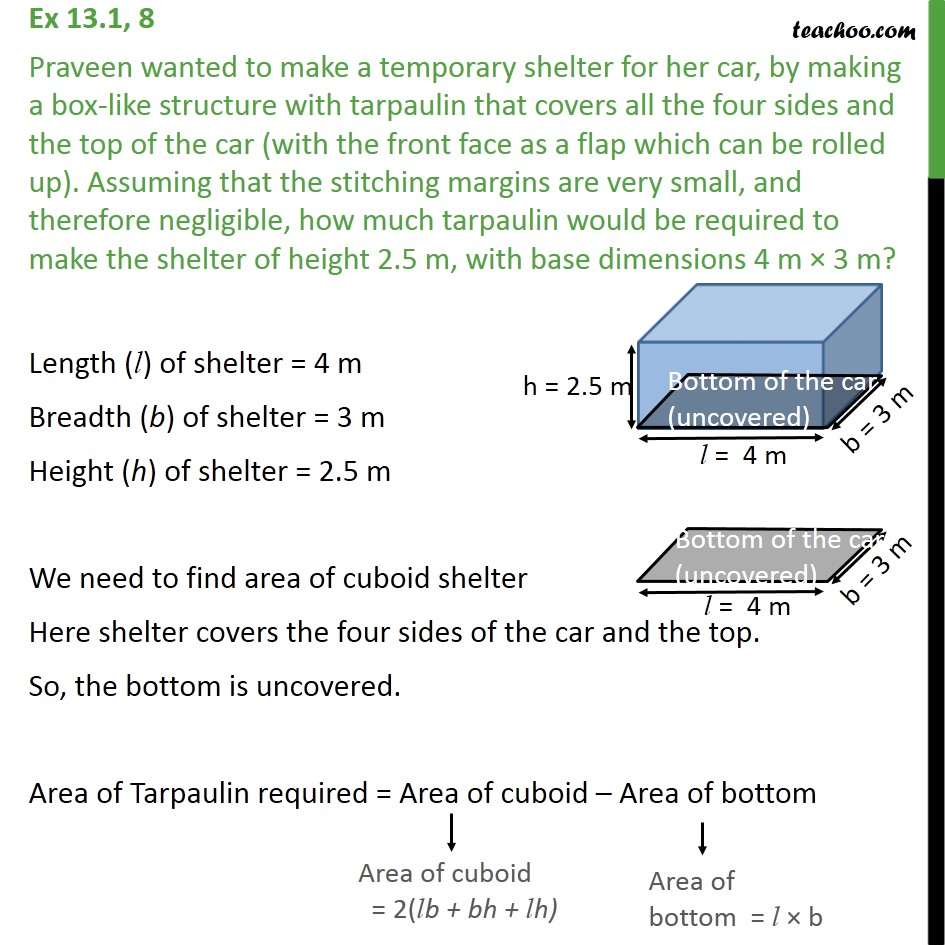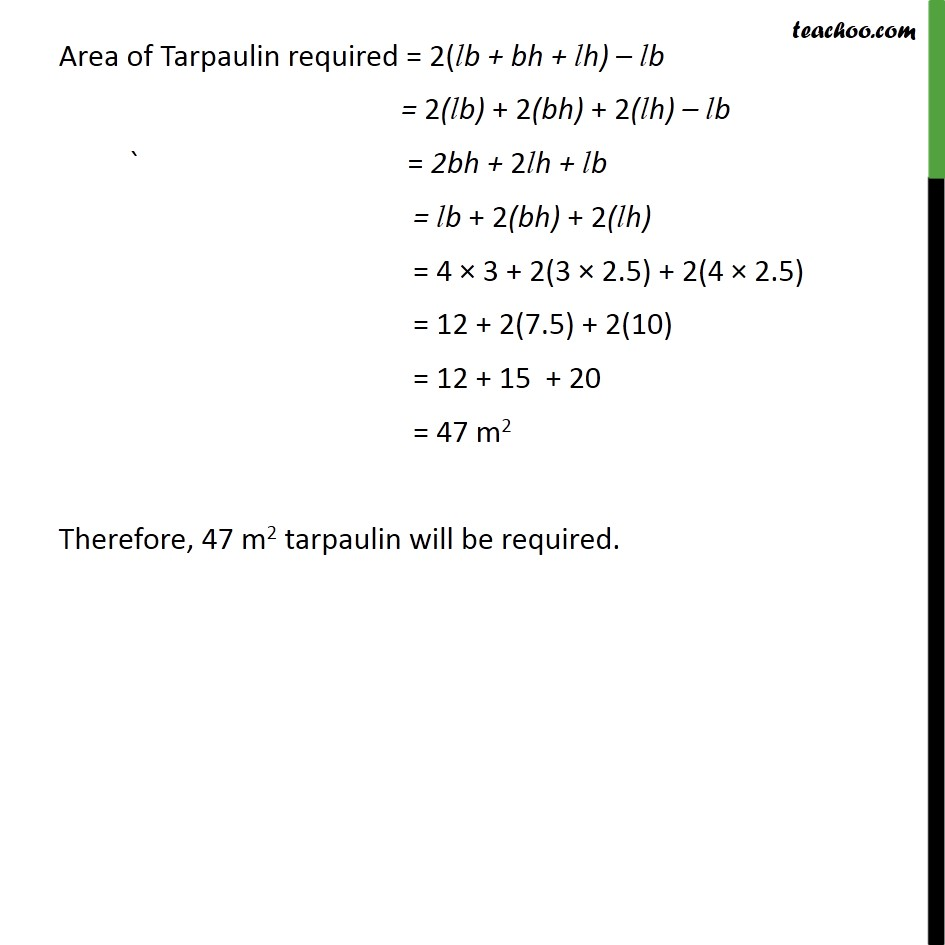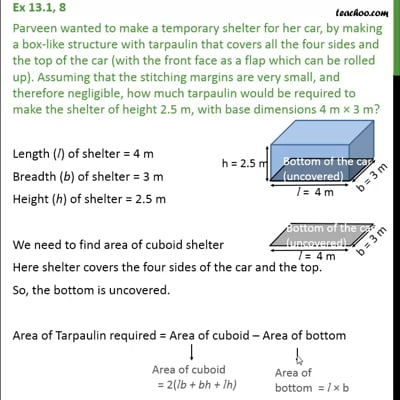Ex 13.1

Chapter 13 Class 9 Surface Areas and Volumes (Term 2)
Serial order wiseThis video is only available for Teachoo black users

### Transcript

Ex 13.1, 8 Praveen wanted to make a temporary shelter for her car, by making a box-like structure with tarpaulin that covers all the four sides and the top of the car (with the front face as a flap which can be rolled up). Assuming that the stitching margins are very small, and therefore negligible, how much tarpaulin would be required to make the shelter of height 2.5 m, with base dimensions 4 m × 3 m? Length (l) of shelter = 4 m Breadth (b) of shelter = 3 m Height (h) of shelter = 2.5 m We need to find area of cuboid shelter Here shelter covers the four sides of the car and the top. So, the bottom is uncovered. Area of Tarpaulin required = Area of cuboid – Area of bottom Area of Tarpaulin required = 2(lb + bh + lh) – lb = 2(lb) + 2(bh) + 2(lh) – lb ` = 2bh + 2lh + lb = lb + 2(bh) + 2(lh) = 4 × 3 + 2(3 × 2.5) + 2(4 × 2.5) = 12 + 2(7.5) + 2(10) = 12 + 15 + 20 = 47 m2 Therefore, 47 m2 tarpaulin will be required.